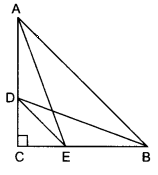# D and E are points on the sides CA and CB respectively of a triangle ABC right angled at C. Prove that $AE^2 + BD^2 = AB^2 + DE^2$.

Given:

D and E are points on the sides CA and CB respectively of a triangle ABC right angled at C.

To do:

We have to prove that $AE^2 + BD^2 = AB^2 + DE^2$.

Solution:In $\triangle \mathrm{ABC}$, $\angle \mathrm{C}=90^{\circ}$

This implies, by Pythagoras theorem,

$\mathrm{AB}^{2}=\mathrm{AC}^{2}+\mathrm{BC}^{2}$.....(i)

In $\triangle \mathrm{DCE}$,

$\mathrm{DE}^{2}=\mathrm{DC}^{2}+\mathrm{CE}^{2}$........(ii)

Adding equations (i) and (ii), we get,

$\mathrm{AB}^{2}+\mathrm{DE}^{2}=\mathrm{AC}^{2}+\mathrm{BC}^{2}+\mathrm{DC}^{2}+\mathrm{CE}^{2}$

$=\mathrm{AC}^{2}+\mathrm{CE}^{2}+\mathrm{DC}^{2}+\mathrm{BC}^{2}$

$=\mathrm{AE}^{2}+\mathrm{BD}^{2}$         (Since $\mathrm{BD}^{2}=\mathrm{DC}^{2}+\mathrm{BC}^{2}$ and $\mathrm{AE}^{2}=\mathrm{AC}^{2}+\mathrm{CE}^{2}$)

Hence proved.